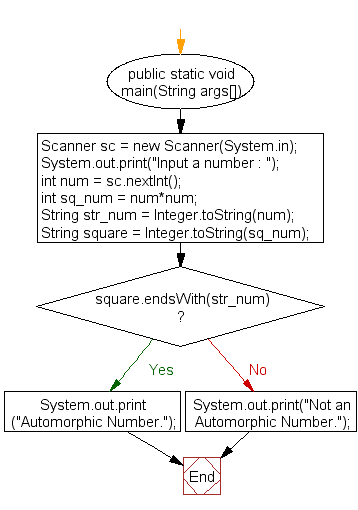﻿ Java exercises: Check whether a number is an Automorphic number or not - w3resource# Java Exercises: Check whether a number is an Automorphic number or not

## Java Numbers: Exercise-14 with Solution

Write a Java program check whether a number is an Automorphic number or not.

In mathematics, an automorphic number is a number whose square "ends" in the same digits as the number itself. For example, 52 = 25, 62 = 36, 762 = 5776, and 8906252 = 793212890625, so 5, 6, 76 and 890625 are all automorphic numbers.

Test Data
Input a number : 76

Pictorial Presentation:Sample Solution:

Java Code:

``````import java.util.Scanner;
public class Example14 {

public static void main(String args[])
{
Scanner sc = new Scanner(System.in);
System.out.print("Input a number : ");
int num = sc.nextInt();
int sq_num = num*num;

String str_num = Integer.toString(num);
String square = Integer.toString(sq_num);

if(square.endsWith(str_num))
System.out.println("Automorphic Number.");
else
System.out.println("Not an Automorphic Number.");
}
}
```
```

Sample Output:

```Input a number : 76
Automorphic Number.
```

Flowchart:Java Code Editor:

What is the difficulty level of this exercise?

﻿

## Java: Tips of the Day

Array vs ArrayLists:

The main difference between these two is that an Array is of fixed size so once you have created an Array you cannot change it but the ArrayList is not of fixed size. You can create instances of ArrayLists without specifying its size. So if you create such instances of an ArrayList without specifying its size Java will create an instance of an ArrayList of default size.

Once an ArrayList is full it re-sizes itself. In fact, an ArrayList is internally supported by an array. So when an ArrayList is resized it will slow down its performance a bit as the contents of the old Array must be copied to a new Array.

At the same time, it's compulsory to specify the size of an Array directly or indirectly while creating it. And also Arrays can store both primitives and objects while ArrayLists only can store objects.

Ref: https://bit.ly/3o8L2KH